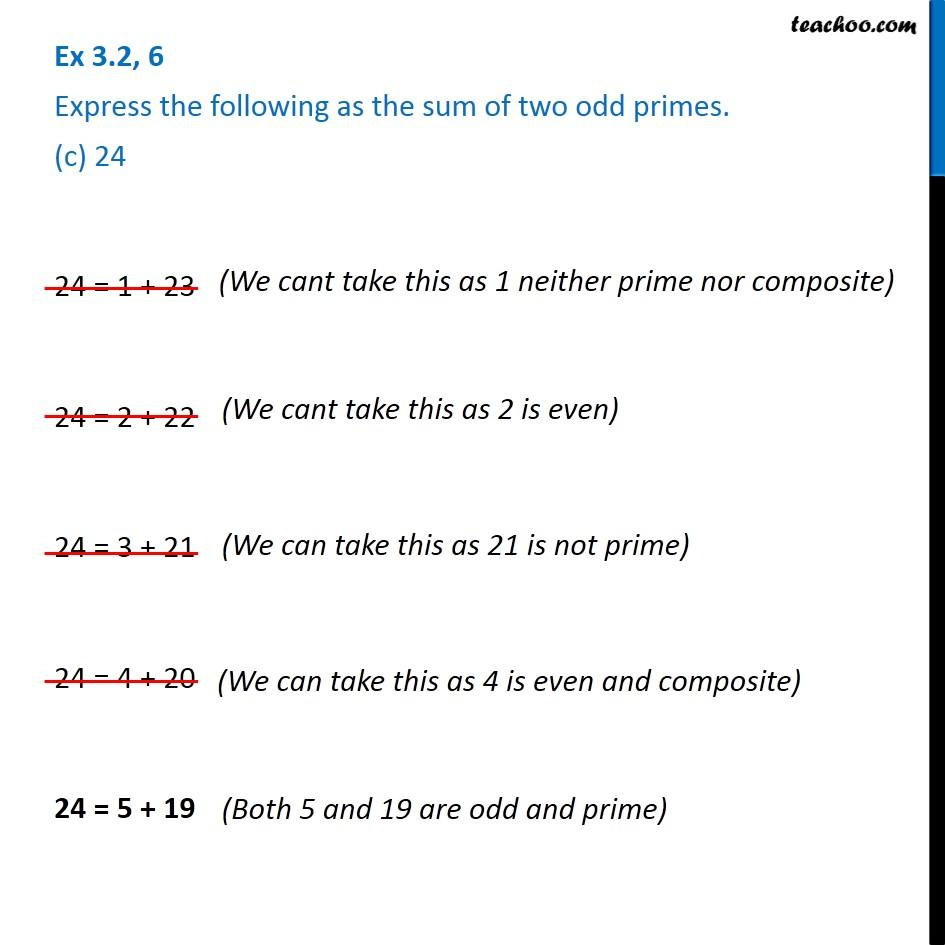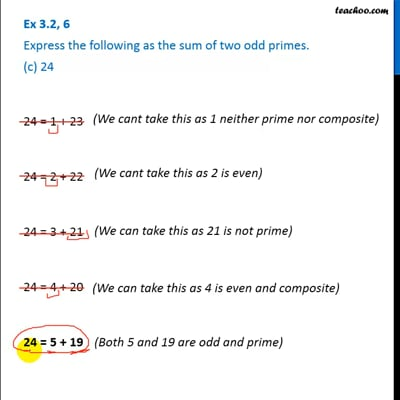Ex 3.2

Chapter 3 Class 6 Playing with Numbers
Serial order wiseThis video is only available for Teachoo black users

Introducing your new favourite teacher - Teachoo Black, at only ₹83 per month

### Transcript

Ex 3.2, 6 Express the following as the sum of two odd primes. (c) 24(We cant take this as 1 neither prime nor composite) (We cant take this as 2 is even) 24 = 1 + 23 24 = 2 + 22 24 = 3 + 21 24 = 4 + 20 24 = 5 + 19 (We can take this as 4 is even and composite) (Both 5 and 19 are odd and prime)